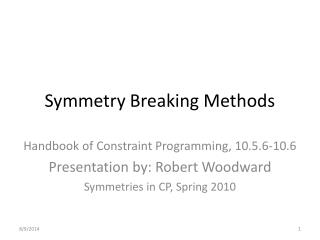DownloadDownload PresentationSymmetry Breaking Methods

# Symmetry Breaking Methods

Download Presentation## Symmetry Breaking Methods

- - - - - - - - - - - - - - - - - - - - - - - - - - - E N D - - - - - - - - - - - - - - - - - - - - - - - - - - -
##### Presentation Transcript

1. Symmetry Breaking Methods Handbook of Constraint Programming, 10.5.6-10.6 Presentation by: Robert Woodward Symmetries in CP, Spring 2010

2. Overview • SBDS & SBDD Review • GE-trees • Definition • Example • STAB Method • Definition • Example • Combination of Methods • Conclusion

3. SBDS & SBDD Review • SBDD (Symmetry breaking via dominance detection) • check at every node in the search tree to see if the node about to be explored is symmetrically equivalent to one already explored • SBDS (Symmetry breaking during search) • add constraints to a problem so that, after backtracking from a search decision, the SBDS constraints ensure that no symmetric equivalent of that decision is ever allowed

4. Overview • SBDS & SBDD Review • GE-trees • Definition • Example • STAB Method • Definition • Example • Combination of Methods • Conclusion

5. GE-trees – Definition • GE = Group Equivalence • GE-tree – Any search tree satisfying: • A tree in which no two nodes are symmetrically equivalent • For every solution to the problem, a symmetrically equivalent node is in the tree • Nodes on the tree represents equivalence classes of partial assignments under the group • Used for value symmetry • Though can also be constructed for variable and mixed variable & value

6. GE-trees – Uses • Intended to classify / compare symmetry breaking techniques • Any method of GE-tree construction will break all problem symmetries as the search for solutions proceed • SBDS and SBDD can be viewed as methods for constructing GE-trees • So can lex-leader (but not subsets)

7. GE-trees – Example • Example: Graph Coloring (Each color indistinguishable) • First node can be colored arbitrarily • Second node must be the same or different • Third node must be same as either of the first two or different • Roney-Dougal et al. used GE-tree paradigm to create a polynomial time algorithm for breaking arbitrary value symmetries • Experiment between GE-trees and GAP-SBDD for problems which only have value symmetry • GE-trees are best in all cases • Reason: GAP-SBDD has potentially exponential search at each node to break symmetry, GE-Trees has low-order polynomial algorithm

8. Overview • SBDS & SBDD Review • GE-trees • Definition • Example • STAB Method • Definition • Example • Combination of Methods • Conclusion

9. STAB Method - Definition • Symmetry Breaking Using Stabilizers • Adds symmetry breaking constraints during search • Only breaks symmetries that leave the partial assignment at a current node unchanged • Breaks symmetry in the stabiliser GA • Stabiliser is a set of the elements of the group that do not change the value of a point when they are applied to the point • Example: stabiliser of 1 is {id, d1} • Is an incomplete method regards to symmetry breaking • Does not return only the non-isomorphic solutions • Can fix by combining SBDD with STAB, which outperforms SBDD alone • So at each assignment it is adding the constraint • V ≤lex Vg, for all g ϵGA

10. STAB Method – Example V: Problem σ: A permutation of the problem A: Partial Assignment Constraint to add: [0,0,0,1,1,0,1,1,0,0,x11,x12,x13,x14,x15,x16,x17,x18,x19,x20] ≤lex [0,0,0,1,1,0,1,1,0,0,x11,x14,x15,x13,x12,x16,x19,x20,x18,x17] Constraint to add: [x11,x12,x13,x14,x15,x16,x17,x18,x19,x20] ≤lex [x11,x14,x15,x13,x12,x16,x19,x20,x18,x17]

11. Overview • SBDS & SBDD Review • GE-trees • Definition • Example • STAB Method • Definition • Example • Combination of Methods • Conclusion

12. Combination of Methods • Each method has its advantages / disadvantages • Difficult to combine methods • What solution each method keeps differs • If care is taken, methods can be combined but results were not good empirically • Puget showed that variable symmetry can be broken by simple ordering constraints, when there is an all-different constraint. • Combined with GE-trees to also break value symmetry • Later, others combined GAP-SBDD and GE-trees • Broke all symmetry, but less efficient than GAP-SBDD alone

13. Overview • SBDS & SBDD Review • GE-Trees • Definition • Example • STAB Method • Definition • Example • Combination of Methods • Conclusion

14. Conclusion • There are a lot of various methods to break symmetry • SBDS • SBDD • GE-trees • STAB • and combinations • They each have their advantages and disadvantages, so there is no one general solution

15. Thank You! • Any questions?